JEE  >  Integer Answer Type Questions: Definite Integrals & Application of Integrals

# Integer Answer Type Questions: Definite Integrals & Application of Integrals - Notes | Study All Types of Questions for JEE - JEE

 1 Crore+ students have signed up on EduRev. Have you?

Q.1. If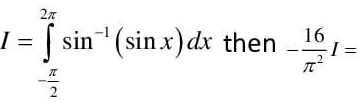Ans. 2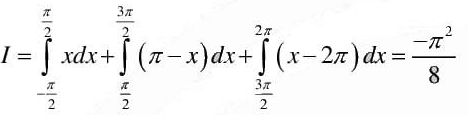Q.2. The value of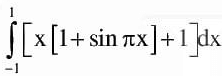is ([.] denote the greatest integer function)

Ans. 2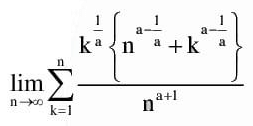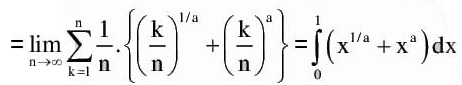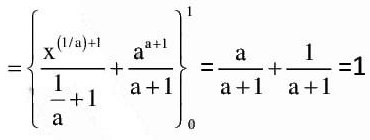Q.3. If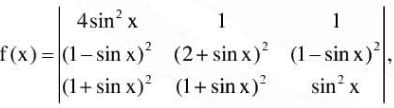then evaluate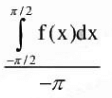Ans. 4
Applying C1→ C1 - C3 and C2→ C2 - C3/ we get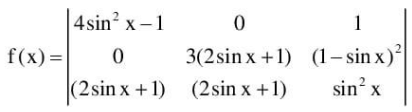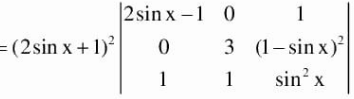= -16sin3x cos2x - 24 sin2x cos2x - 12 sinx cos2x - 2 cos2x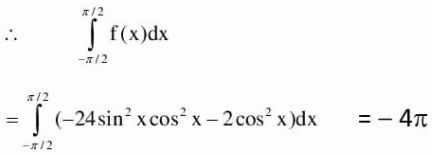Q.4.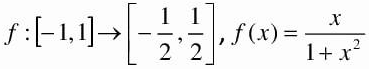If the area bounded by y = f-1 (x) , x-axis between, x = -1/2 to x = 1/2 is A - In 2, then find A .

Ans. 1
Required area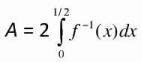Let f-1 (x)= t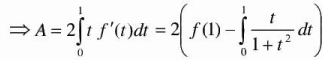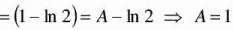Q.5. Let y = g(x) be the image of f(x) = x + sinx a bout the line x + y = 0. If the a rea bounded by y = g(x), x-axis, x = 0 and x = 2 tt is A, then A/π2 is ___________

Ans. 2
f(x) is an odd function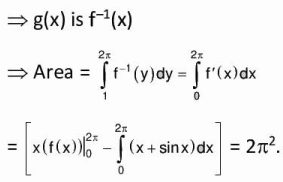The document Integer Answer Type Questions: Definite Integrals & Application of Integrals - Notes | Study All Types of Questions for JEE - JEE is a part of the JEE Course All Types of Questions for JEE.
All you need of JEE at this link: JEE

## All Types of Questions for JEE

376 docs
 Use Code STAYHOME200 and get INR 200 additional OFF

## All Types of Questions for JEE

376 docs

Track your progress, build streaks, highlight & save important lessons and more!

,

,

,

,

,

,

,

,

,

,

,

,

,

,

,

,

,

,

,

,

,

;